# Assignment 4: Investigating Importance Sampling

## Due: Tuesday May 16th, 11:59PM

Questions? Need help? Post them on the Assignment4Discussion page so everyone else can see!

## Description

Rendering requires the computation of many different types of integrals. In the last assignment we numerically integrated over the back element of the realistic camera lens to compute the irradiance indicent on each pixel of the camera's film. As you are now well aware, the variance of a monte carlo estimator manifests itself as noise in a rendered image. Thus, when integrating a function, it is important to choose samples from the domain of integration such that variance in the integral estimate is minimized. Importance sampling is a variance reduction technique that draws samples using a distribution that is proportional to the value of the function over the domain. In this assignment you will explore various approaches for sampling irradiance on scene objects due to an infinite area light source (environment light) and explain when certain approaches are preferrable to others.

We are happy to inform you that this assignment is designed to be significantly shorter than Homework 2 and 3.

Read chapters 13 (especially 13.5), 14 (especially 14.3.4) and 15 (especially 15.6) in the pbrt book. This assignment will require a solid understanding of importance sampling, so please read the these chapters carefully.

Additionally, you may want to take a closer look at the Monte Carlo integration your performed in assignment 3. Read this document to see why.

## Step 2: Importance Sampling an Infinite Area Light

In the scenes we've rendered in this class so far, objects were lit using a small number of spotlight or area light sources. In the real world, light incident on an object comes not just from light emitters, but from all directions. One way of approximating this effect in pbrt is to use an environment light (infinite area light in pbrt), which is used to define light entering the scene from all directions due the surrounding environment. Environment lighting can greatly contribute to the realism and richness of your renderings, however, since computing environment lighting involves integrating incoming radiance over the entire hemisphere, in the worst case, Monte Carlo estimates are prone to be noisy, and require many samples to produce images of reasonable quality. In the scene below, images of the killeroo scene are rendered with 4, 8, and 128 samples per pixel.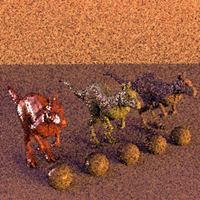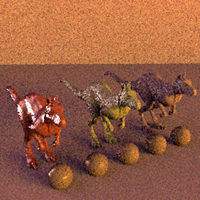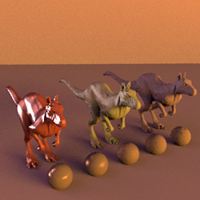Importance sampling the environment map can make an enormous difference in the quality of the rendered image. Both images below are rendered using the same number of samples. In the image below at left, samples are drawn from a cosine-weighted distribution centered about the normal of the surface being shaded (see lights/infinite.cpp). At right, samples are drawn using a probability distribution that is proportional to the luminance of the environment map (see lights/infinitesample.cpp).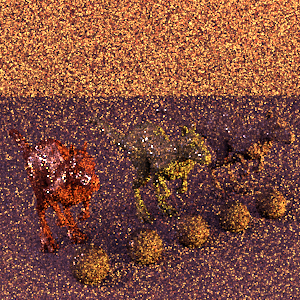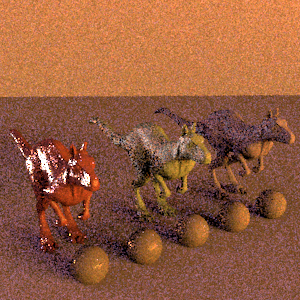Question 1. As an exercise, assume that you are given an environment map with luminance L(phi, theta) = cos(theta)  for theta > PI/2 and L(phi, theta) = 0 otherwise. Write down a pdf that could be used to sample the environment with respect to luminance. Use the inversion method to find expressions for phi and theta in terms of uniform random variables x1 and x2 so that a direction on the sphere is chosen randomly according to your pdf.

Note in simple cases such as this, integrating the pdf analytically is possible. In practice, radiance from each position is defined by a texture map and the pdf is determined numerically. This is precisely what is done by lights/infinitesample.cpp.

## Step 3: Multiple Importance Sampling

When computing reflected surface radiance due to direct lighting, pbrt computes a Monte Carlo estimate of the integral of incoming light over all directions modulated by the surface's BSDF. To improve the quality of this estimate, it is desirable to use importance sampling when choosing a direction in which to sample indicent radiance. The integrand consists of two terms, one related to the surface BSDF and the second related to radiance from light sources, and pbrt samples incoming radiance by drawing samples according to pdfs associated with each of the terms. This technique is called multiple importance sampling (MIS). It is implemented in pbrt in the file core/transport.cpp.

1. We would like to you add support for a 'sample' parameter to the 'directlighting' integrator that allows you to select between using the default importance sampling implementation, or sampling only according to either the light's or BSDF's pdf. Possible values of this parameter are 'bsdf', 'light', and 'mis'. This parameter controls whether the samples are drawn from BSDF, light source, or both distributions. Set the default value to be 'mis'. When you disable MIS, make sure that your estimate of direct lighting remains unbiased. (How might you verify this?)

2. The scene mis.pbrt defines the scene that is shown on page 677 of pbrt. Render mis.pbrt with each of the 3 sampling schemes. You should get images similar those on page 676 in the textbook. Be sure that you render all scenes with the same number of samples (Careful: the number of samples specified in the .pbrt file may need to change so that the same number of samples are taken when MIS is disabled).

Question 2. When sampling according to the BSDF, some regions of the checkboard are rendered with little noise, and in other regions noise is severe. Describe which is which, and explain with this occurs when sampling according to the BSDF.

Question 3. Provide a similar explanation for the results you see when sampling with respect to the light sources. Are the bad cases when sampling with respect to the BSDF the same as the bad cases when sampling with respect to the lights?

Question 4. The image produced using multiple importance sampling exhibits the least amount of noise. How does this approach solve the problems you discussed in Questions 2 and 3?

## Step 4: Exploring Importance Sampling in the Killeroo Scene

Before proceeding with this step, you'll need to build lights/infinitesample.cpp into a shared object called infinitesample.dll. You modify the project or Makefiles from assignment 3 to do this.

Render killeroo_view1.pbrt with grace_latlong.exr as the environment map texture using the following settings. This scene contains three killeroos, ranging in specularity from most speculat at left to least specular at right. In front of the killeroos are a set of spheres of varying specularity. Use a total of 16 samples light source during your direct lighting estimate (note this may mean you specify a different number when using MIS)

• Light samples, BSDF only distribution,
• Light only distribution (using infinitesample as the light)
• Using multiple importance sampling (with infinitesample as the light).

Question 5. Discuss parts of the image that look better when using BSDF sampling than when using sampling based on environment map luminance.

Question 6. Notice that overall, the image is less noisy when using environment map sampling than using multiple importance sampling. Is this also the case when rendering using the skylight-sunset.exr environment map? Explain what is going on here based on your understanding of how samples in lights/infinitesample.cpp are generated, and the content of the scene and the environment maps.

Question 7. We have seen that using environment map luminance as a pdf for importance sampling can significantly reduce the variance in rendered images. Is this always true? Describe a situation that this sampling strategy may not be as effective as the cosine-weighted sampling implemented in lights/infinite.cpp. Try and construct a scene that demonstrates this (using light only sampling in this experiment).

## Step 5: Short Questions

1) Explain why for the same scene setup and same number of samples,

• the killeroo image with 'grace_latlong' environment map look more noisy than the one with the 'sunset' envrionment map.